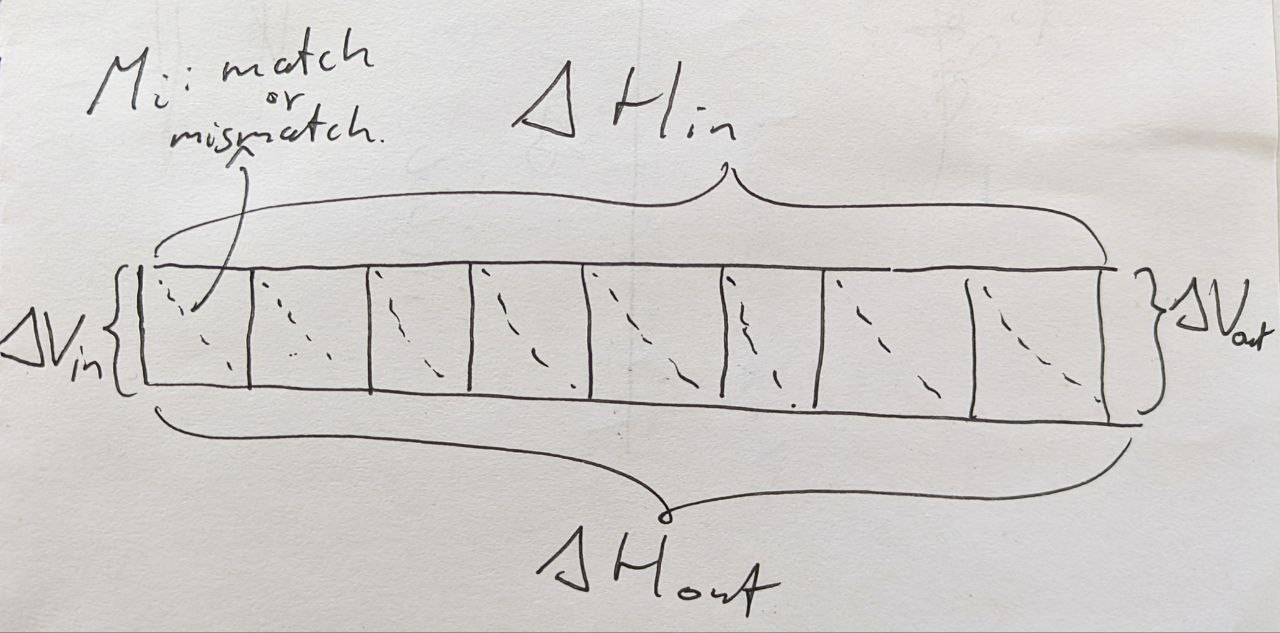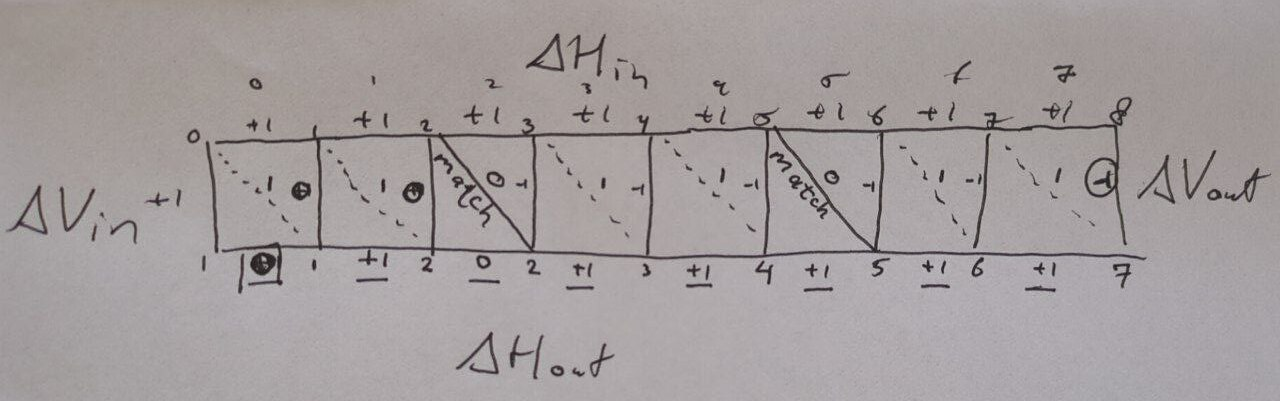# BitPAl bitpacking algorithm

The supplement (download) of the Loving, Hernandez, and Benson (2014) paper introduces a $$15$$ operation version of Myers (1999) bitpacking algorithm, which uses $$16$$ operations when modified for edit distance.

I tried implementing it, but it seems to have a bug that I will describe below. It may well be that the bug is in my understanding of the code rather than the code itself.

## Problem

To recap, this algorithm solves the unit-cost edit distance problem by using bitpacking to compute a $$1\times w$$ at a time. As input, it takes

• horizontal differences (each in $$\{-1, 0, +1\}$$) along the top,
• the vertical differences on the left (also in $$\{-1,0,+1\}$$),
• which characters along the top match the character on the left.

It outputs:

• the new horizontal differences along the bottom,
• the new vertical difference on the right.Figure 1: Bitpacking layout of BitPAl, showing a row of (w=8) cells.

## Input

• A bitvector $$M$$ indicating for $$w$$ horizontally adjacent positions whether there is a match. $$M_i = 1$$ when the $$i$$’th cell contains a match. I’ll use $$w=8$$ here.
• A bitvector $$D$$, with $$D_i = 1$$ when the horizontal difference along the top of cell $$i$$ is Decreasing, i.e. $$-1$$.
• A bitvector $$SD$$ ($$S|D$$ in that paper), with $$SD_i = 1$$ when the horizontal difference along the top of cell $$i$$ is the Same or Decreasing, i.e. $$0$$ or $$-1$$.
• The vertical difference on the left is assumed to be $$1$$ in case of edit distance.

## Example

Consider the following example input:

• All horizontal input differences are $$+1$$, i.e. $$\Delta H_in = (+1,+1,\dots,+1)$$. This means none are decreasing or the same, so $$D=00000000_2$$ and $$SD=00000000_2$$ (in binary).
• The vertical input difference is also $$+1$$, as already assumed for edit distance.
• There are matches at positions $$2$$ and $$5$$, so $$M = (0,0,1,0,0,1,0,0) = 00100100_2$$.Figure 2: Example with horizontal and vertical input deltas all equal to (1) and some matches (solid diagonal lines).

Working through the example (Figure 2), we see that the output horizontal differences are $$(0, 1, 0, 1, 1, 1, 1, 1)$$, so we expect $$D = 0$$ (no decreases) and $$SD = 00000101_2$$ (the first position corresponds to the least significant digit).

Now let’s run through the code.

1. $$M_m = 00100100 | 0 = 00100100$$

2. $$R_{I|S} = \sim M_m = 11011011$$

3. $$notM_I = R_{IS} | SD = R_{IS} | 0 = 11011011$$

(Note, the paper writes $$R_{IS}$$ here but $$R_{I|S}$$ on the line above.)

4. $$R_{I|S}orS = notM_I \wedge D = notM_I \wedge 0 = 11011011$$

5. $$Sum = notM_I + SD = 11011011 + 0 = 11011011$$

6. $$MaskSum = Sum \& R_{I|S} = 11011011 \& 11011011 = 11011011$$

7. $$V_0 = MaskSum \wedge R_{I|S}orS = 11011011$$

8. $$V_{+1} = D | (MaskSum \& SD) = 0 | (11011011 \& 0) = 0$$

9. $$V_0^{\ll} = V_0 \ll 1 = 0$$

10. $$V_1^{\ll} = V_1 \ll 1 = 0$$

11. $$V_1^{\ll} = V_1^\ll + 1 = 00000001$$

12. $$D = M_m \& V_{+1}^\ll = 00100100 \& 00000001 = 0$$

13. $$SD = V_{+1}^\ll | (M_m \wedge V_0^\ll) = 00000001 | (00100100 \& 0) = 00000001$$

We see that this gives a different result $$SD = 00000001_2$$ instead of $$SD = 00000101_2$$.

Looking closer, after step $$7$$, $$V_0$$ should indicate the cells with vertical difference $$0$$ on their right side, ie the first two cells or $$00000011_2$$, but instead its value is $$11011011$$.

## Discussion

I’m not exactly sure what’s wrong, but I think things already fail before the summation, since currently no carry happens at all. A carry is expected because it is the only step that introduces long-range dependencies between bit-positions.

Maybe I have some fundamental misunderstanding of the meaning of the input or output parameters, but the text accompanying the code seems to agree with my understanding. On the other hand, I did not fully understand the brief explanation regarding the runs of ones and how they are resolved by the summation step.

Loving, Joshua, Yozen Hernandez, and Gary Benson. 2014. “Bitpal: A Bit-Parallel, General Integer-Scoring Sequence Alignment Algorithm.” Bioinformatics 30 (22): 3166–73. https://doi.org/10.1093/bioinformatics/btu507.
Myers, Gene. 1999. “A Fast Bit-Vector Algorithm for Approximate String Matching Based on Dynamic Programming.” Journal of the Acm 46 (3): 395–415. https://doi.org/10.1145/316542.316550.